Word Mover's Distance (WMD) is a distance metric used to measure the dissimilarity between two documents, and its application in text analytics was introduced by a research group from Washington University in 2015. The group's paper, From Word Embeddings To Document Distances, was published on the 32nd International Conference on Machine Learning (ICML). In this paper, they demonstrated that the WMD metric leads to unprecedented low k-nearest neighbor document classification error rates on eight real world document classification data sets.

They leveraged word embedding and WMD to classify documents, and the biggest advantage of this method over the traditional method is its capability to incorporate the semantic similarity between individual word pairs (e.g. President and Obama) into the document distance metric. In a traditional way, one method to manipulate semantically similar words is to provide a synonym table so that the algorithm can merge words with same meaning into a representative word before measuring document distance, otherwise you cannot get an accurate dissimilarity result. However, maintaining synonym tables need continuous efforts of human experts and thus is time consuming and very expensive. Additionally, the semantic meaning of words depends on domain, and the general synonym table does not work well for varied domains.

### Definition of Word Mover's Distance

WMD is the distance between the two documents as the minimum (weighted) cumulative cost required to move all words from one document to the other document. The distance is calculated through solving the following linear program problem.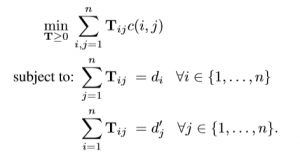Where

• Tij denotes how much of word i in document d travels to word j in document d';
• c(i; j) denotes the cost “traveling” from word i in document d to word j in document d'; here the cost is the two words' Euclidean distance in the word2vec embedding space;
• If word i appears ci times in the document d, we denote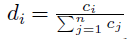WMD is a special case of the earth mover's distance metric (EMD), a well-known transportation problem.

### How to Calculate Earth Mover's Distance with SAS?

SAS/OR is the tool to solve transportation problems. Figure-1 shows a transportation example with four nodes and the distances between nodes, which I copied from this Earth Mover's Distance document. The objective is to find out the minimum flow from {x1, x2} to {y1, y2}. Now let's see how to solve this transportation problem using SAS/OR.

The weights of nodes and distances between nodes are given below.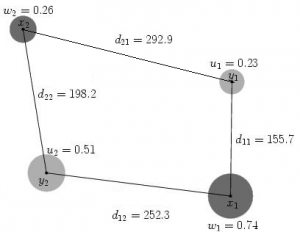Figure-1 A Transportation Problem

```data x_set; input _node_ \$ _sd_; datalines; x1 0.74 x2 0.26 ;   data y_set; input _node_ \$ _sd_; datalines; y1 0.23 y2 0.51 ;   data arcdata; input _tail_ \$ _head_ \$ _cost_; datalines; x1 y1 155.7 x1 y2 252.3 x2 y1 292.9 x2 y2 198.2 ;   proc optmodel; set xNODES; num w {xNODES};   set yNODES; num u {yNODES};   set &lt;str,str&gt; ARCS; num arcCost {ARCS};   read data x_set into xNODES=[_node_] w=_sd_; read data y_set into yNODES=[_node_] u=_sd_; read data arcdata into ARCS=[_tail_ _head_] arcCost=_cost_;   var flow {&lt;i,j&gt; in ARCS} &gt;= 0; impvar sumY = sum{j in yNODES} u[j]; min obj = (sum {&lt;i,j&gt; in ARCS} arcCost[i,j] * flow[i,j])/sumY;   con con_y {j in yNODES}: sum {&lt;i,(j)&gt; in ARCS} flow[i,j] = u[j]; con con_x {i in xNODES}: sum {&lt;(i),j&gt; in ARCS} flow[i,j] &lt;= w[i];   solve with lp / algorithm=ns scale=none logfreq=1; print flow; quit;```

The solution of SAS/OR as Table-1 shows, and the EMD is the objective value: 203.26756757.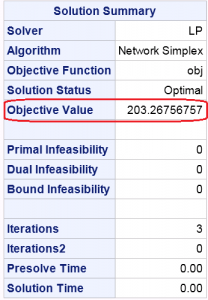Table-1 EMD Calculated with SAS/OR

The flow data I got with SAS/OR as Table-2 shows the following, which is same as the diagram posted in the aforementioned Earth Mover's Distance document.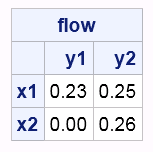Table-2 Flow data of SAS/OR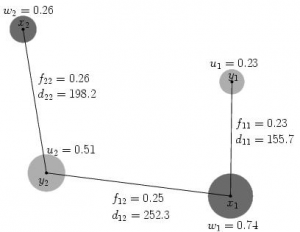Figure-2 Flow Diagram of Transportation Problem

### How to Calculate Word Mover's Distance with SAS

The paper, From Word Embeddings To Document Distances, proposed a new metric called Relaxed Word Mover's Distance (RWMD) by removing the second constraint of WMD in order to decrease the calculations. Since we need to read word embedding data, I will show you how to calculate RWMD of two documents with SAS Viya.

```/* start CAS server */ cas casauto host="host.example.com" port=5570; libname sascas1 cas;   /* load documents into CAS */ data sascas1.documents; infile datalines delimiter='|' missover; length text varchar(300); input text\$ did; datalines; Obama speaks to the media in Illinois.|1 The President greets the press in Chicago.|2 ; run;   /* create stop list*/ data sascas1.stopList; infile datalines missover; length term \$20; input term\$; datalines; the to in ; run;   /* load word embedding model */ proc cas; loadtable path='datasources/glove_100d_tab_clean.txt' caslib="CASTestTmp" importOptions={ fileType="delimited", delimiter='\t', getNames=True, guessRows=2.0, varChars=True } casOut={name='glove' replace=True}; run; quit;   %macro calculateRWMD ( textDS=documents, documentID=did, text=text, language=English, stopList=stopList, word2VectDS=glove, doc1_id=1, doc2_id=2 ); /* text parsing and aggregation */ proc cas; textParse.tpParse/ table = {name="&amp;textDS",where="&amp;documentID=&amp;doc1_id or &amp;documentID=&amp;doc2_id"} docId="&amp;documentID", language="&amp;language", stemming=False, nounGroups=False, tagging=False, offset={name="outpos", replace=1}, text="&amp;text"; run;   textparse.tpaccumulate/ parent={name="outparent1", replace=1} language="&amp;language", offset='outpos', stopList={name="&amp;stoplist"}, terms={name="outterms1", replace=1}, child={name="outchild1", replace=1}, reduce=1, cellweight='none', termWeight='none'; run; quit;   /* terms of the two test documents */ proc cas; loadactionset "fedsql"; execdirect casout={name="doc_terms",replace=true} query=" select outparent1.*,_term_ from outparent1 left join outterms1 on outparent1._termnum_ = outterms1._termnum_ where _Document_=&amp;doc1_id or _Document_=&amp;doc2_id; " ; run; quit;     /* term vectors and counts of the two test documents */ proc cas; loadactionset "fedsql"; execdirect casout={name="doc1_termvects",replace=true} query=" select word2vect.* from &amp;word2VectDS word2vect, doc_terms where _Document_=&amp;doc2_id and lowcase(term) = _term_; " ; run;   execdirect casout={name="doc1_terms",replace=true} query=" select doc_terms.* from &amp;word2VectDS, doc_terms where _Document_=&amp;doc2_id and lowcase(term) = _term_; " ; run;   simple.groupBy / table={name="doc1_terms"} inputs={"_Term_", "_Count_"} aggregator="n" casout={name="doc1_termcount", replace=true}; run; quit;   proc cas; loadactionset "fedsql"; execdirect casout={name="doc2_termvects",replace=true} query=" select word2vect.* from &amp;word2VectDS word2vect, doc_terms where _Document_=&amp;doc1_id and lowcase(term) = _term_; " ; run;   execdirect casout={name="doc2_terms",replace=true} query=" select doc_terms.* from &amp;word2VectDS, doc_terms where _Document_=&amp;doc1_id and lowcase(term) = _term_; " ; run;   simple.groupBy / table={name="doc2_terms"} inputs={"_Term_", "_Count_"} aggregator="n" casout={name="doc2_termcount", replace=true}; run; quit;   /* calculate Euclidean distance between words */ data doc1_termvects; set sascas1.doc1_termvects; run;   data doc2_termvects; set sascas1.doc2_termvects; run;   proc iml; use doc1_termvects; read all var _char_ into lterm; read all var _num_ into x; close doc1_termvects;   use doc2_termvects; read all var _char_ into rterm; read all var _num_ into y; close doc2_termvects;   d = distance(x,y);   lobs=nrow(lterm); robs=nrow(rterm); d_out=j(lobs*robs, 3, ' '); do i=1 to lobs; do j=1 to robs; d_out[(i-1)*robs+j,1]=lterm[i]; d_out[(i-1)*robs+j,2]=rterm[j]; d_out[(i-1)*robs+j,3]=cats(d[i,j]); end; end;   create distance from d_out; append from d_out; close distance; run;quit;   /* calculate RWMD between documents */ data x_set; set sascas1.doc1_termcount; rename _term_=_node_; _weight_=_count_; run;   data y_set; set sascas1.doc2_termcount; rename _term_=_node_; _weight_=_count_; run;   data arcdata; set distance; rename col1=_tail_; rename col2=_head_; length _cost_ 8; _cost_= col3; run;   proc optmodel; set xNODES; num w {xNODES};   set yNODES; num u {yNODES};   set &lt;str,str&gt; ARCS; num arcCost {ARCS};   read data x_set into xNODES=[_node_] w=_weight_; read data y_set into yNODES=[_node_] u=_weight_; read data arcdata into ARCS=[_tail_ _head_] arcCost=_cost_;   var flow {&lt;i,j&gt; in ARCS} &gt;= 0; impvar sumY = sum{j in yNODES} u[j]; min obj = (sum {&lt;i,j&gt; in ARCS} arcCost[i,j] * flow[i,j])/sumY;   con con_y {j in yNODES}: sum {&lt;i,(j)&gt; in ARCS} flow[i,j] = u[j]; /* con con_x {i in xNODES}: sum {&lt;(i),j&gt; in ARCS} flow[i,j] &lt;= w[i];*/   solve with lp / algorithm=ns scale=none logfreq=1;   call symput('obj', strip(put(obj,best.))); create data flowData from [i j]={&lt;i, j&gt; in ARCS: flow[i,j].sol &gt; 0} col("cost")=arcCost[i,j] col("flowweight")=flow[i,j].sol; run; quit;   %put RWMD=&amp;obj; %mend calculateRWMD;   %calculateRWMD ( textDS=documents, documentID=did, text=text, language=English, stopList=stopList, word2VectDS=glove, doc1_id=1, doc2_id=2 );```

The RWMD value is 5.0041121662.

Now let's have a look at the flow values.

```proc print data=flowdata; run;quit;```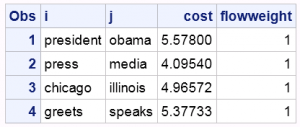WMD method does not only measure document similarity, but also it explains why the two documents are similar by visualizing the flow data.

Besides document similarity, WMD is useful to measure sentence similarity. Check out this article about sentence similarity and you can try it with SAS.

Share